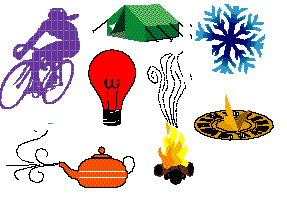# Forces of Nature

#### Lab Assignment: Elastic CollisionsForces of Nature Lab

# Kinetic Energy and Momentum in Elastic Collisions

## Goal: To measure the changes in momentum and energy during a collision.

Ideally, you would do this with pucks on an air table (such as the kind used for air hockey). If you don't have access to one, use the instructions below to create "ice pucks" to use on a glass surface.

#### Materials

1. Pendulum
• Fixed point for attaching string.
• String (measure length!)
• Bob of known mass; should be equal to or less than the mass of the ice pucks.
• Ruler to measure height to which you raise bob.
2. Six tuna cans or other cylindrical mold
3. Distilled water
4. Glass plate, large mirror, or other flat, very smooth surface
5. Cardboard barriers to run around the edge of the flat surface, except where the pendulum bob will hit the puck.
6. Stop watch, video camera, or other device to record puck motion and measure time.

#### Procedures

1. Set up the pendulum and glass surface as shown in the diagram.
2. Puck Creation.
• Fill each tuna can with the same amount of distilled water and freeze it, making sure that the cans are set on a level surface.
• When you are ready to perform the experiment, take out the number of cans you need for that experiment. Set each briefly in a pan of hot water to loosen the "ice pucks" and pop them from the cans. If necessary to get a smooth sliding surface, partially melt one of the circular sides until it is flat.
• Weigh each ice puck and record its weight.
3. Wipe the glass surface clean.
4. Pendulum-single puck collision.• Based on the masses of the puck and bob, predict the collision behavior of both. Will the bob bounce back? Will the puck move?
• Raise the pendulum bob so that when you let go, it will collide with the puck. Record puck elevation, then let go.
• Repeat your experiment using different starting heights for the bob.
• If possible, record the time it takes the ice puck to cross your glass plate or a fixed distance.
• Record the behavior of the puck and pendulum.
5. Puck collision• Based on the masses of the bob and both pucks, predict the behavior when the bob mass hits the first puck, and when the first puck hits the second puck.
• Perform the collision, recording the height of the pendulum bob.

#### Data Analysis

1. Create a table that contains columns for bob mass, pendulum height, potential energy stored in the pendulum, puck mass, velocity of pendulum puck after collision, velocity of ice puck after collision. If there is a second puck involved, include columns for its mass and post-collision velocity.
2. Include one final column with a conclusion about whether your observations support the calculated velocities.

#### Data Analysis

Create a table of your data. You may use a spreadsheet to perform calculations. If you carry out the experiment several times with different masses, arrange your masses in increasing order and plot selected data as a function of mass changes.

• Record the mass of the bob in kilograms.
• Record the height the bob in kilograms for each trial
• Calculate the initial potential energy for the bob.
• Calculate the maximum velocity of the bob at its lowest point.
• Record the mass of the puck.
• Record the time required for the puck to travel a set distance after the collision.
• Compute the kinetic energy transfered to the puck when struck.
• Record the bounce-back height of the pendulum.
• Compute the potential energy retained by the pendulum bob.
• Compute the change in momentum for the pendulum bob and the puck.
• Determine whether your observations and computations are reasonable (within experimental error)

Use a similar method to record observational data for the two-puck experiment.

#### Report

Follow the standard format for reporting your experiment. Be sure to include your data, sample calculations, and conclusions.